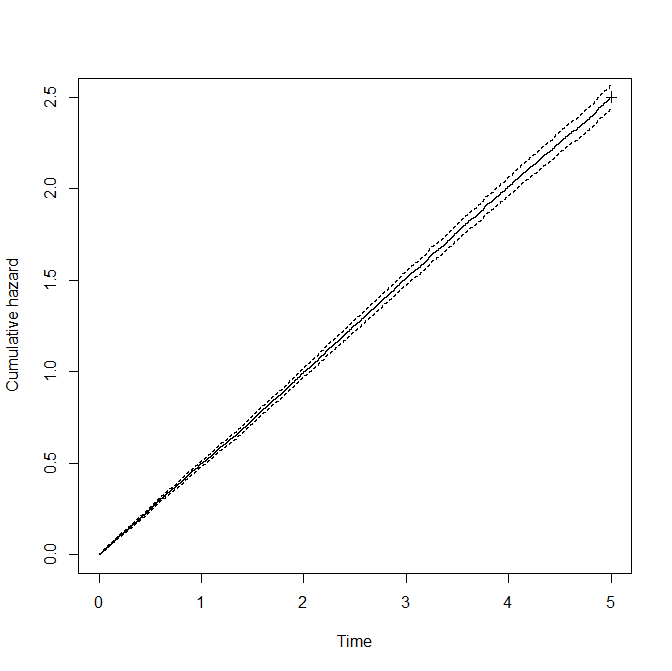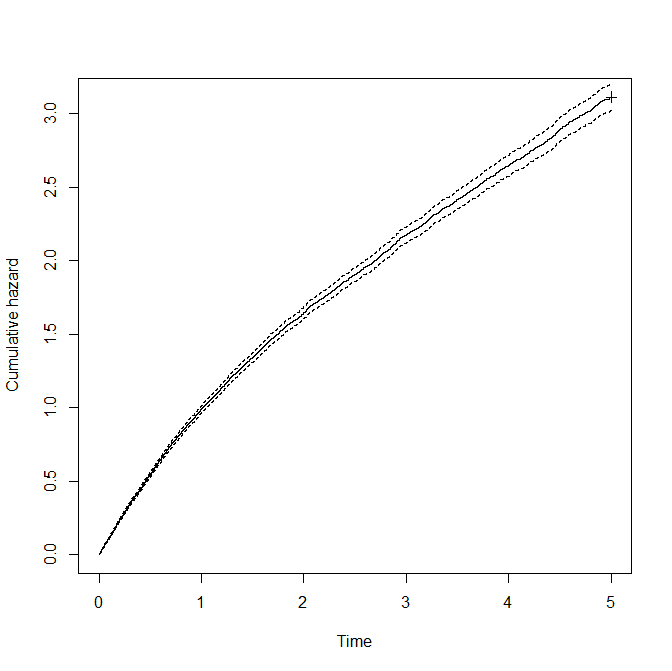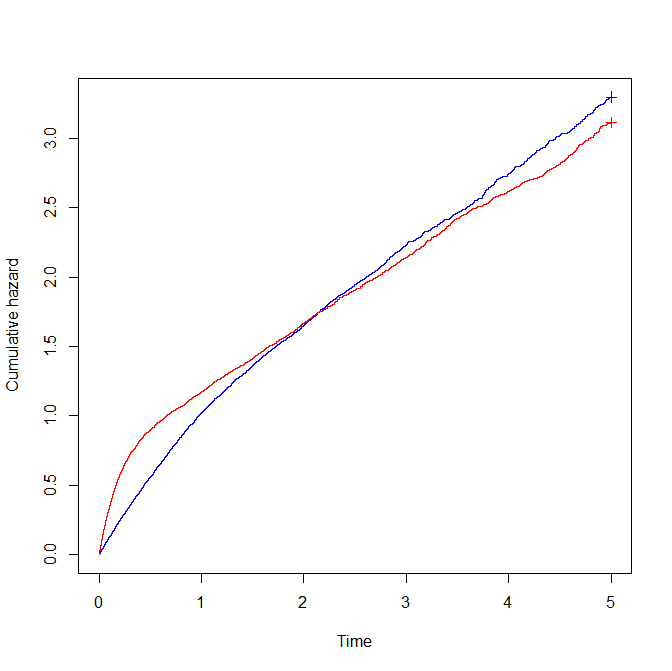# Interpreting changes in hazard and hazard ratios

I recently attended a great course by Odd Aalen, Ornulf Borgan, and Hakon Gjessing, based on their book Survival and Event History Analysis: a process point of view. Among the many interesting topics covered was the issue of how to interpret changes in estimated hazard functions, and similarly, changes in hazard ratios comparing two groups of subjects.

The hazard function
In survival (or more generally, time to event) analysis, the hazard function $h(t)$ at a time $t$ specifies the instantaneous rate at which subject's experience the event of interest, given that they have survived up to time $t$:

$h(t) = \lim_{\delta t \rightarrow 0} \frac{P(t \leq T < t+\delta t|T\geq t)}{\delta t}$

where $T$ denotes the random variable representing the survival time of a subject. Perhaps the most common plot used with survival data is the Kaplan-Meier survival plot, of the function $S(t)=P(T>t)$. To illustrate, let's simulate some survival data in R:

n <- 10000
h <- 0.5
t <- -log(runif(n))/h


This code simulates survival times where the hazard function $h(t)=0.5$, i.e. a constant. One of the special feature of survival data is that often the survival times are censored. In our simulation we will create a very simple censoring mechanism in which survival times are censored at $t=5$:

event <- 1*(t<5)
obstime <- t
obstime[obstime>=5] <- 5


Now let's plot the estimated survival function, using the survival package in R:

survfit <- survfit(Surv(obstime,event) ~ 1)
plot(survfit, ylab="Survival probability", xlab="Time")


This gives:

The 95% confidence interval limits are very close to the estimated line here because we have simulated a dataset with a large sample size.

We can see here that the survival function is not linear, even though the hazard function is constant. This is because the two are related via:

$S(t) = \exp(-H(t))$

where $H(t) = \int^{t}_{0} h(u) du$ denotes the cumulative hazard function. In our setup $H(t)=0.5t$, so that the true survival function equals $S(t) = \exp(-0.5t)$.

The cumulative hazard function
To see whether the hazard function is changing, we can plot the cumulative hazard function $H(t)$, or rather an estimate of it:

plot(survfit, fun="cumhaz", ylab="Cumulative hazard", xlab="Time")


which gives:Here we can see that the cumulative hazard function is a straight line, a consequence of the fact that the hazard function is constant.

Changing hazards
Sometimes the hazard function will not be constant, which will result in the gradient/slope of the cumulative hazard function changing over time. The obvious interpretation is that the hazard being experienced by individuals is changing with time. However, as we will now demonstrate, there is an alternative, sometimes quite plausible, alternative explanation for such a phenomenon.

We will now simulate survival times again, but now we will divide the group into 'low risk' and 'high risk' individuals. The low risk individuals will again have (constant) hazard equal to 0.5, but the high risk subjects will have (constant) hazard 2:

highrisk <- 1*(runif(n) < 0.5)
h <- 0.5+highrisk*1.5
t <- -log(runif(n))/h
event <- 1*(t<5)
obstime <- t
obstime[obstime>=5] <- 5


Once again, we plot the cumulative hazard function:

survfit <- survfit(Surv(obstime,event) ~ 1)
plot(survfit, fun="cumhaz", ylab="Survival probability", xlab="Time")


which gives:Cumulative hazard plot where sample consists of 50% low risk and 50% high risk subjects
The natural interpretation of this plot is that the hazard being experienced by subjects is decreasing over time, since the gradient/slope of the cumulative hazard function is decreasing over time. However, we are in the fortunate position here that we know how the survival data are generated. We know that the sample consists of 'low risk' and 'high risk' subjects, who have time constant hazards of 0.5 and 2 respectively. Why then does the cumulative hazard plot suggest that the hazard is decreasing over time? Because as time progresses, more of the high risk subjects are failing, leaving a larger and larger proportion of low risk subjects in the surviving individuals. Since the low risk subjects have a lower hazard, the apparent hazard is decreasing.

This difficulty or issue with interpreting the hazard function arises because we are implicitly assuming that the hazard function is the same for all subjects in the group. When, as will often be the case, the hazard varies between subjects, we may see the hazard changing because of so called 'selection effects' - the high risk individuals (on average) fail early, such that the remaining subjects have, on average, lower hazard than the hazard of the group at $t=0$.

Changing hazard ratios
The same issue can arise in studies where we compare the survival of two groups, for example in a randomized trial comparing two treatments. Such a comparison is often summarised by estimating a hazard ratio between the two groups, under the assumption that the ratio of the hazards of the two groups is constant over time, using Cox's proportional hazards model. In some studies it is seen that the hazard ratio changes over time. Again the 'obvious' interpretation of such a finding is that effect of one treatment compared to the other is changing over time.

However, from our analysis above we can see that such a result could also arise through selection effects. For example, suppose again that the population consists of 'low risk' and 'high risk' subjects, and that we randomly assign two treatments to a sample of 10,000 subjects. We will assume the treatment has no effect on the low risk subjects, but that for high subjects it dramatically increases the hazard:

highrisk <- 1*(runif(n)<0.5)
treat <- 1*(runif(n)<0.5)
h <- 0.5+highrisk*(1.5+6*treat)
t <- -log(runif(n))/h
event <- 1*(t<5)
obstime <- t
obstime[obstime>=5] <- 5


Let's now plot the cumulative hazard function, separately by treatment group:

survfit <- survfit(Surv(obstime,event) ~ 1+treat)
plot(survfit, fun="cumhaz", col=c(4,2), ylab="Cumulative hazard", xlab="Time")


which gives:Cumulative hazard function. Red corresponds to the treat=1 group, and blue to the treat=0 group
The interpretation of this plot is that the treat=1 group (in red) initially have a higher hazard than the treat=0 group, but that later on, the treat=1 group has a lower hazard than the treat=0 group. That is, the hazard ratio comparing treat=1 to treat=0 is greater than one initially, but less than one later. We might interpret this to mean that the new treatment initially has a detrimental effect on survival (since it increases hazard), but later it has a beneficial effect (it reduces hazard).

However, based on the mechanism we used to generate the data, we know that the treatment has no effect on low risk subjects, and has a detrimental effect (at all times) for high risk subjects. Again what we see is as a result of selection effects. In the treat=1 group, the 'high risk' subjects have a greatly elevated hazard (manifested in the steeper cumulative hazard line initially), and thus they die off rapidly, leaving a large proportion of low risk patients at the later times. In contrast, in the treat=0 group, a larger proportion of high risk patient remain at the later times, such that this group appears to have greater hazard than the treat=1 group at later times.

Of course in reality we do not know how data are truly generated, such that if we observed changing hazards or changing hazard ratios, it may be difficult to work out what is really going on. In a nice paper published in Epidemiology, Miguel Hernan explains the selection effect issue which afflicts the hazard function (and hazard ratios) and discusses the Women's Health Initiative as an example of a study where the hazard ratio changes over time.

Some alternatives
Given the preceding issues with interpreting changes in hazards or hazard ratios, what might we do? In the clinical trial context, the simple Kaplan-Meier plot can of course be used. When it is desired to present a single measure of a treatment's effects, we could use the difference in median (or some other appropriate percentile) survival time between groups.

In an observational study there is of course the issue of confounding, which means that the simple Kaplan-Meier curve or difference in median survival cannot be used. To overcome this Hernan suggests the use of adjusted survival curves, constructed via discrete time survival models. In their book, Aalen, Borgan and Gjessing describe how to construct adjusted survival curves based on Aalen's additive hazard regression modelling approach.

A further alternative is to fit so called frailty models, which explicitly model between subject variability in hazard via random-effects. A difficulty however in the case of survival data is that such models are only identifiable if one is willing to make assumptions about the shape of the hazard function. Without making such assumptions, we cannot really distinguish between the case where between-subject variability exists in hazards from the case of truly time-changing individual hazards.

For more about this topic, I'd recommend both Hernan's 'The hazard of hazard ratios' paper and Chapter 6 of Aalen, Borgan and Gjessing's book.

This site uses Akismet to reduce spam. Learn how your comment data is processed.